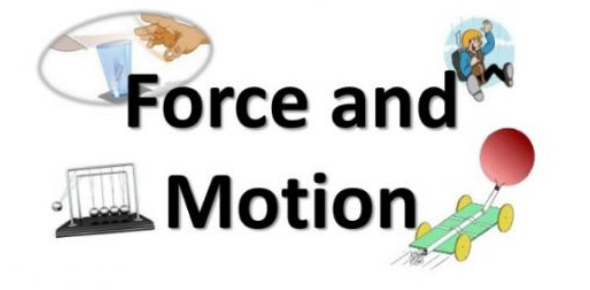# Force And Motion Practice Test Quiz! Trivia

47 Questions | Total Attempts: 673SettingsForce and motion practice test quiz trivia! Force is energy exerted over an object which has the ability to change its motion from one point to another. Force and motion help in making things move or stop. There are basically two types of forces, which include distance force and contact forces. This quiz will help you review what you know about forces and their effect on motion. Give it a try!

• 1.
Forces can _______________ an object.
• A.

Stop the motion of

• B.

Change the mass of

• C.

Change the smell of

• D.

Change the colour of

• 2.
Which of the following is NOT a type of force?
• A.

Gravitational

• B.

Magnetic

• C.

Heat

• D.

Weight

• 3.
What is the SI unit of force?
• A.

Kilogram

• B.

Centimetre

• C.

Joules

• D.

Newton

• 4.
Which of the following action involves a push and a pull?
• A.

Dragging a chair

• B.

Punching a punching bag

• C.

Lifting a box

• D.

Turning a steering wheel

• E.

All of the above..

• 5.
It would probably be easiest to ride a bicycle across
• A.

Gravel

• B.

Sand

• C.

Grass

• D.

• 6.
The force that always acts opposite to the direction of the motion of an object is called
• A.

Reaction

• B.

Inertia

• C.

Friction

• D.

Lift

• 7.
Which is NOT a force?
• A.

Mass

• B.

Weight

• C.

Gravity

• D.

Air resistance

• 8.
The following helps to reduce the friction when moving a cabinet EXCEPT
• A.

Moving on a rough surface

• B.

Putting lubricant on the surface

• C.

Removing the content in the cabinet

• D.

Placing wheels for the cabinet

• 9.
The amount of force exerted on an object due to gravity is the
• A.

Mass

• B.

Weight

• C.

Upthrust

• D.

Inertia

• 10.
Which of the following is a contact force?
• A.

Static electricity

• B.

Air resistance

• C.

Magnetic force

• D.

Gravity

• 11.
The amount of matter in an object is the
• A.

Weight

• B.

Gravity

• C.

Mass

• D.

• 12.
The tendency of an object to remain at rest if at rest or to continue moving in a straight line if in motion is called
• A.

Friction

• B.

Inertia

• C.

Force

• D.

Acceleration

• 13.
Your weight changes on different planets. Which is the most important factor?
• A.

The distance away from the Sun

• B.

The size of the planet (mass)

• C.

The density of the planet

• D.

The magnetic effect of the planet

• 14.
Which of the following metals is NOT magnetic?
• A.

Iron

• B.

Cobalt

• C.

Aluminium

• D.

Nickel

• 15.
What is a magnetic field?
• A.

An object that loses its magnetism after a short time.

• B.

A magnet's ability to attract certain metals.

• C.

The space around a magnet where the force of a magnet can act.

• D.

The areas of a magnet where its force of attraction is strongest.

• 16.
In a bar magnet, the strongest magnetic force is
• A.

In the middle

• B.

At the North pole

• C.

At the South pole

• D.

At both poles

• 17.
You can magnetise a nail by
• A.

Stroking the nail with a magnet in one direction for 30 times

• B.

Heating the nail for 2 minutes

• C.

Moving the nail to and fro on the bar magnet

• D.

Using a sand paper to polish the nail

• 18.
Which of the following is CORRECT?
• A.

North pole attracts north pole

• B.

South pole attracts north pole

• C.

North pole repels south pole

• D.

South pole attracts south pole

• 19.
What is the term used to represent the micro-magnets in a magnetic substance?
• A.

Domino

• B.

Domains

• C.

Remains

• D.

Magnetlets

• 20.
The following set up is used to build
• A.

Heated nail

• B.

Magnetic coils

• C.

An electromagnet

• D.

A permanent magnetic

• 21.
What is a frame of reference?
• A.

An ability to change the size of motion

• B.

Object or background from which movement is compared

• C.

A thing that rotates with the sun

• D.

Object or background which is compared with another object

• 22.
Looking at the Pacific Ocean, when the sun drops below the horizon of California is the sun falling or are you moving?
• A.

The sun is falling

• B.

You are moving

• 23.
Change in position in a certain amount of time is called __________.
• 24.
When you describe motion, you are comparing it with some frame of reference.
• A.

True

• B.

False

• 25.
The rate at which an object moves is __________.
Related TopicsBack to top